Test: Struts & Columns - 3

# Test: Struts & Columns - 3

Test Description

## 10 Questions MCQ Test Topicwise Question Bank for Mechanical Engineering | Test: Struts & Columns - 3

Test: Struts & Columns - 3 for Mechanical Engineering 2023 is part of Topicwise Question Bank for Mechanical Engineering preparation. The Test: Struts & Columns - 3 questions and answers have been prepared according to the Mechanical Engineering exam syllabus.The Test: Struts & Columns - 3 MCQs are made for Mechanical Engineering 2023 Exam. Find important definitions, questions, notes, meanings, examples, exercises, MCQs and online tests for Test: Struts & Columns - 3 below.
Solutions of Test: Struts & Columns - 3 questions in English are available as part of our Topicwise Question Bank for Mechanical Engineering for Mechanical Engineering & Test: Struts & Columns - 3 solutions in Hindi for Topicwise Question Bank for Mechanical Engineering course. Download more important topics, notes, lectures and mock test series for Mechanical Engineering Exam by signing up for free. Attempt Test: Struts & Columns - 3 | 10 questions in 30 minutes | Mock test for Mechanical Engineering preparation | Free important questions MCQ to study Topicwise Question Bank for Mechanical Engineering for Mechanical Engineering Exam | Download free PDF with solutions
 1 Crore+ students have signed up on EduRev. Have you?
Test: Struts & Columns - 3 - Question 1

### Compression members always tend to buckle in the direction of the

Test: Struts & Columns - 3 - Question 2

### Euler’s formula gives 5 to 10% error in crippling load as compared to experimental results in practice because

Detailed Solution for Test: Struts & Columns - 3 - Question 2

Euler's formula to calculate crippling load in the column

$${P_{cr}} = \frac{{{\pi ^2}EI_{mini}}}{{L_e^2}}$$

Where P = Crippling load, E = young's modulus, Imini = Minimum area moment of inertia of column, Le = Effective length of a column

The assumption made while developing Euler Formula for the crippling load of a column does not meet in the real-life practice due to which therefore Euler's formula gives 5 to 10 % error compared to experimental results or true value.

The following assumptions are made in Euler's column theory :

• The column is initially perfectly straight and the load is applied axially.
• The cross-section Of the column is uniform throughout its length.
• The column material is perfectly elastic, homogeneous, and isotropic and obeys Hooke's law.
• The length of the column is very large as compared to its lateral dimensions.
• The direct stress is very small as compared to the bending stress.
• The self-weight of the column is negligible.
• The column will fail by buckling alone.
• Pin joints are free from friction.
Test: Struts & Columns - 3 - Question 3

### Match List-I (Long column) with List-ll (Critical Load) and select the correct answer using the codes given below the lists: List-I A. Both ends hinged B. One end fixed and other end free C. Both ends fixed D. One end fixed and other end hinged List-II 1. π2 EI/4L2 2. 4π2 EI/4L2 3. 2π2 EI/4L2 4. π2 EI/L2 Codes:     A B C D (a) 2 1 4 3 (b) 4 1 2 3 (c) 2 3 4 1 (d) 4 3 2 1

Detailed Solution for Test: Struts & Columns - 3 - Question 3

(i) Both ends hinged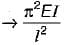(ii) One end fixed and other end free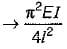(iii) Both ends fixed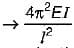(iv) One end fixed and other end hinged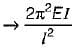Test: Struts & Columns - 3 - Question 4

Given that
PE = the crippling load given by Euler
Pc = the load at failure due to direct compression
PR = the load in accordance with the Rankine’s criterion of failure
Then PR is given by

Detailed Solution for Test: Struts & Columns - 3 - Question 4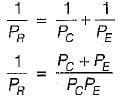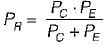Test: Struts & Columns - 3 - Question 5

If the crushing stress in the material of a mild steel column is 3300 kg/cm2. Euler’s formula for crippling load is applicable for slenderness ratio equal to/greater than

Detailed Solution for Test: Struts & Columns - 3 - Question 5

For Euler’s formula to be applicable the critical stress must not exceed the proportional limit.
Now crushing stress in mild steel
= 3300 kg/cm2
= 330 N/mm2
But stress at proportional limit in mild steel
= 250 M/mm2
Euler’s buckling stress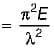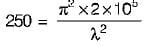λ = 88.55 ≈ 89
Thus slenderness ratio should be more than or equal to 89 ideally. Option (d) is the most close one.

Test: Struts & Columns - 3 - Question 6

For a circular column having its ends hinged, the slenderness ratio is 160. The L/d ratio of the column is

Detailed Solution for Test: Struts & Columns - 3 - Question 6

Slenderness ratio,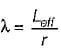where r is the least radius of gyration and Leff is effective length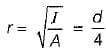∴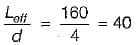Test: Struts & Columns - 3 - Question 7

When a column is subjected to an eccentric load, the stress induced in the column will be

Test: Struts & Columns - 3 - Question 8

An aluminium column of square cross-section (10 mm x 10 mm) and length 300 mm has both ends pinned. This is replaced by a circular cross-section (of diameter 10 mm) column of the same length and made of the same material with the same end conditions. The ratio of critical stresses for these two columns corresponding to Euler ’s critical load (σcritical) square : (σcritical)circular is

Detailed Solution for Test: Struts & Columns - 3 - Question 8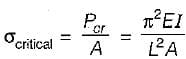For same material, same end condition and same length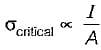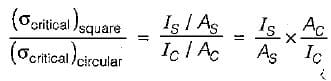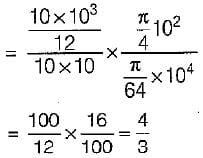Test: Struts & Columns - 3 - Question 9

If one end of a hinged column is made fixed and the other free, how much is the critical load compared to the original value?

Detailed Solution for Test: Struts & Columns - 3 - Question 9

Original load =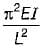when one end of hinged column is fixed and other free. New Lθ = 2L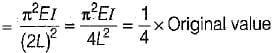Test: Struts & Columns - 3 - Question 10

A short column of external diameter D and internal diameter d carries and eccentric load W. The greatest eccentricity which the load can be applied without producing tension on the cross-section of the column would be

Detailed Solution for Test: Struts & Columns - 3 - Question 10

Direct stress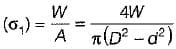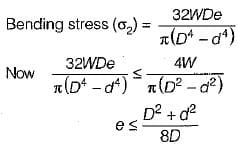## Topicwise Question Bank for Mechanical Engineering

58 videos|314 tests
 Use Code STAYHOME200 and get INR 200 additional OFF Use Coupon Code
Information about Test: Struts & Columns - 3 Page
In this test you can find the Exam questions for Test: Struts & Columns - 3 solved & explained in the simplest way possible. Besides giving Questions and answers for Test: Struts & Columns - 3, EduRev gives you an ample number of Online tests for practice

## Topicwise Question Bank for Mechanical Engineering

58 videos|314 tests